# Talk:11-1100/Homework Assignment 1

Is this just another way of asking to show that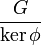$\frac{G}{\ker \phi}$ is normal in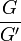$\frac{G}{G'}$? The wording of this question is a little unclear to me.
Answer. More fully, you have to show that given any group homomorphism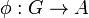$\phi:G\to A$, where$A$ is an Abelian group, there exists a group homomorphism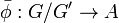$\bar\phi:G/G'\to A$ such that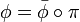$\phi=\bar\phi\circ\pi$, where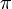$\pi$ is the projection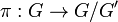$\pi:G\to G/G'$. (Sorry it took me long to respond, I was out of commission for much of last week and I'm only now getting better). Drorbn 09:34, 2 October 2011 (EDT)